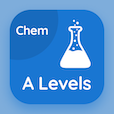A Level Courses Online

A Level Chemistry Prep Tests

A Level Chemistry Tests

The Book Equilibrium Constant Expression Multiple Choice Questions (MCQ Quiz), Equilibrium Constant Expression quiz answers PDF to learn online courses, a level chemistry tests. Study Equilibrium Multiple Choice Questions and Answers (MCQs), Equilibrium Constant Expression quiz questions for online college classes. The eBook Equilibrium Constant Expression MCQ App Download: acid base equilibria, reversible reactions, chemical industry equilibria test prep for SAT prep classes.

The MCQ: Law relating to concentrations of substances present at equilibrium and to the stoichiometric equation is known as PDF, "Equilibrium Constant Expression" App Download (Free) with law of mass action, charle's law, law of conversation of energy, and boyle's law choices for online college classes. Practice equilibrium constant expression quiz questions, download Amazon eBook (Free Sample) for online associates degree.

## Chemistry: Equilibrium Constant Expression MCQs

MCQ: Law relating to concentrations of substances present at equilibrium and to the stoichiometric equation is known as

A) Law of mass action
B) Charle's Law
C) Law of conversation of energy
D) Boyle's law

MCQ: In our blood, hemoglobin is bound to oxygen in an

A) equilibrium expression
B) equilibrium relation
C) equilibrium reactions
D) equilibrium constant

MCQ: The simple relationship between equilibrium concentrations of reactants and products with stoichiometry is called as

A) equilibrium expression
B) equilibrium relation
C) dynamic equilibrium
D) equilibrium constant

MCQ: First calculations on equilibrium were carried out by

A) Cato Guldberg only
B) Peter Waage only
C) John Dalton
D) Cato Guldberg and Peter Waage

### Practice Tests: A Level Chemistry Exam Prep

Download A level Chemistry Quiz App, 10th Grade Chemistry MCQs App and SAT Chemistry MCQ App for Android & iOS devices. These Apps include complete analytics of real time attempts with interactive assessments. Download Play Store & App Store Apps & Enjoy 100% functionality with subscriptions!